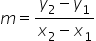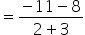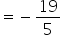Mathematics
Easy

Question

# Find the slope of the line that passes through (-3, 8) and (2, -11)

## -19/513/23/1011/2Hint:

## The correct answer is: -19/5

### Points are (-3, 8) and (2, -11)Step 1 : Slope of a line that passes through (x1,y1 ), (x2, y2 ) is, is the slope of given line ., where ( x1 , y1 ) and ( x2 , y2 ) are the given points and m is the slope .

### Related Questions to study#### With Turito Foundation.#### Get an Expert Advice From Turito.